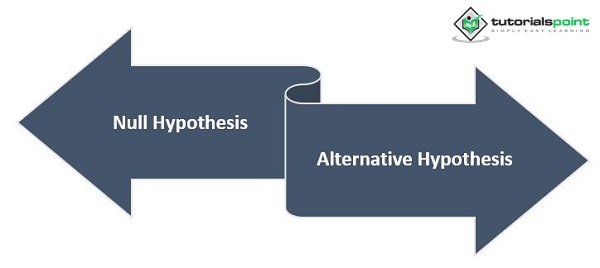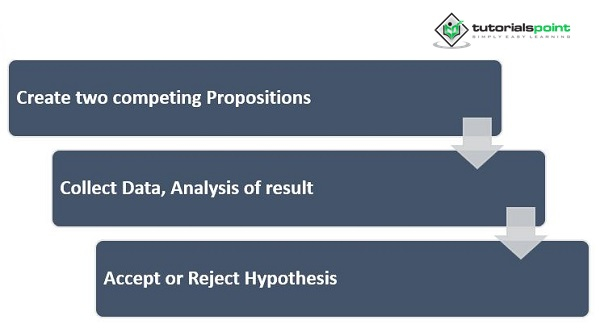# Role of Hypothesis Testing in Psychology

In our lives, we make so many assumptions. These assumptions guide us toward some theory or generalization. In the field of science, these assumptions lead to big discoveries later. For instance, the great inventor Isaac Newton first observed an apple falling from a tree. He must have made certain guesses about the possible causes before discovering the concept of gravity. Similarly, these guesses must have been backed by some evidence. Without sufficient evidence, assumptions remain mere guesses.

## What is the definition of a Hypothesis?

When we talk about a hypothesis, it refers to a tentative statement that can be tested to check its validity or reliability. This means that it is not just any other statement. This tentative proposition is based on some observations and has a good amount of evidence to back it up. We can say that hypotheses have the potential to be proved or disproved, and this implies that a hypothesis has a sure answer

## Where is a Hypothesis used?

The hypothesis is used in many places. People use hypothetical thoughts in their daily conversations. However, hypotheses are frequently used in statistics. When we want to collect and analyze data, hypotheses are very useful. It also helps in putting the information in tabular format. In research, hypotheses are an important element. In summary, the meaning of the hypothesis is as follows

• A possible and tentative statement that explains an event or set of data

• The origin of a hypothesis can come from observation or common sense.

• A hypothesis does not always need to be true. It can be proven incorrect through testing.

• Hypothesis tries to look for connections in the given data.

• It should be noted that a hypothesis is not one person's vision, opinion, or thought; rather, it is a medium through which research can be developed. Hypotheses should not be taken as the final statement; rather, it is considered the means through which a phenomenon or data can be explained scientifically.

## What is Hypothesis Testing?

When researchers have some theory, they come up with a tentative proposition or assumption, known as a hypothesis. Testing the hypothesis refers to the process where the researcher puts the assumption to the test using statistical methods. The method depends on the type of data and the research study the researcher adapts. Testing is conducted to check a hypothesis's credibility with the help of sample information. This sample data is gathered from a larger population, and the sample is representative of that population. There are statistical tools present to gather data

## How is Hypothesis Testing done?

When a researcher wants to test a hypothesis, a sample is chosen using statistical methods. The researcher aims to provide credible evidence to prove the null hypothesis or alternative as plausible. A researcher uses the random sampling method to eliminate biases from the study and increase the credibility of the hypothesis.

## What are the Null Hypothesis and the Alternative Hypothesis?

When a researcher forms a hypothesis, he comes up with two contrasting and competing statements. This ensures they are not leaning toward one side or making definite assumptions. Two competing claims ensure the researcher is open to an alternative possibility contrary to his proposition. A null hypothesis is an assumption that there is no effect of x on the population. The null hypothesis assumes a position of neutrality and thus states that the effect is equal to zero. On the other hand, an alternative hypothesis is an assumption that there is an effect of x on the population. It is the opposite of the null hypothesis, suggesting that the effect is not neutral or equal to zero.When a hypothesis is tested, both possibilities cannot be true simultaneously. This means that either the null or the alternative hypothesis can be true at one point. However, one of the hypotheses is always true.

## What are the Steps in Hypothesis Testing?

Once the researcher has made some observations, they come up with tentative statements or propositions. Here are the next steps they will follow to test their hypothesis.• Create two competing propositions: The researcher states two contrasting hypotheses (null and alternative). This is done so that either one of them is proven.

• The next step involves determining how the hypothesis can be tested. This is done once the data is collected and an analysis method is used to examine the data.

• The final step is to understand and analyze the data closely, and this step helps the researcher understand whether to accept or reject the alternative hypothesis.

## An Example of Hypothesis Testing

If a person observes that engagement in sports boosts the quality of life, they may first form two competing statements. The null hypothesis is that engagement in sports has no impact on the quality of life, and an alternative hypothesis would be that engagement in sports boosts the quality of life. The null hypothesis is represented as Ho, and the alternative hypothesis is represented as Ha.

The researcher would operationally define all the variables present in the study. Later, a sample will be selected randomly, representing the population. The sample is then divided into an experimental group (to whom the treatment is given) and a control group (to whom the treatment is not given). Later, appropriate tests are given to the participants to measure their quality of life. Questionnaires or scales can be used for this purpose. Once the results arrive, the researcher analyzes them closely using several methods. The score is tested for statistical significance. The researcher accepts or rejects the alternative hypothesis based on the results.

## Conclusion

Researchers make several tentative assumptions in order to prove their ideas or theories. A hypothesis is a tentative proposition the researcher makes in favor of their theory. The assumption cannot be taken seriously unless it is tested. In order to prove its credibility, the researcher employs several methods to test its hypothesis. This formal method for examining ideas about various phenomena is called hypothesis testing. With hypothesis testing, it is easier to trust the predictions. These tests also help determine the likelihood of the interrelation between variables arising by chance.